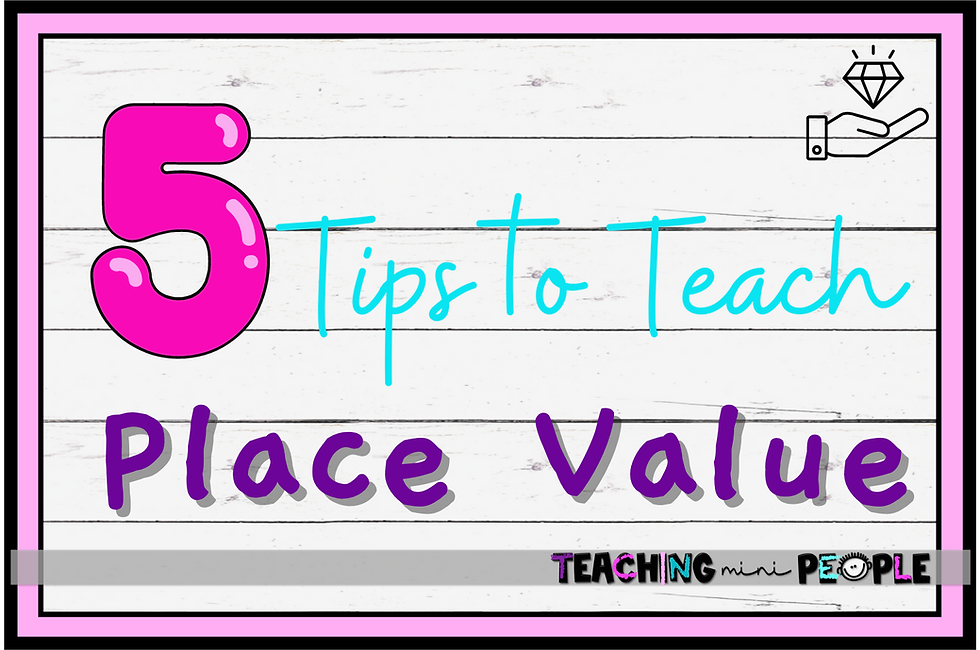top of page
Search
• Kristy Johnson

# 5 Tips for Teaching Place ValueTeaching place value is one of the most important units in upper elementary school, because this is the foundation for all other units. It is important that we take our time teaching this unit to make sure our students have a strong understanding of place value. With this strong understanding, students will be better prepared for each unit that follows. Here are my top five tips for teaching place value in upper elementary.Place Value Chart

First, it is important to talk about and reteach the place value chart and what students learned in the years prior. For example, in fourth grade, students will come in having practice with the ones, the tens, and the hundreds places. So you are going to want to review the place value chart with those three places and then move on to the thousands, ten thousands, hundred thousands, millions, etc.

Once you have retaught the place value chart and students know where each place is, it is then important to teach the difference between each column on the chart. Students not only need to understand where those numbers fall, but also what those numbers mean in each place. Here is where I talk about expanded form and what those numbers look like when stretched out. This not only shows students the zeros behind those numbers, but also shows them why they fall where they do on the place value chart.

### Zeros are Important

Second, students need to understand just how important zero is in a number. Many times teachers will teach zero, as just a placeholder with no meaning; however, this is not true. Although zero means there is nothing there, students need to understand that zero does have meaning, and it is more than just a placeholder. To correct this misconception, if students were taught that way, I will show students what would happen to numbers if that zero wasn’t there. For example, in the number 25,902, if we got rid of the zero we would only have 2,592. That zero is showing us there are no “singles” in the tens place because in that same number we could say we have 50 tens.

### Tie in Multiplication

In third grade, students should start learning their multiplication facts. This also includes multiplying single digits by multiples of ten. Therefore, while teaching place value, it is the perfect time to tie in multiplication. Students must understand that each digit to the left is 10 times bigger than the digit to the right. So using this understanding of multiplication, we can then show our students what the value of each number really is as we move from 1 to 10 to 100 to 1000 and so on.### Place Value Centers

Using centers in my math class is my biggest tip for all units! This stands true in my place value unit as well. Allowing students to practice with place value in a variety of ways will strengthen their knowledge of the content. My favorite type of center is task cards. For my place value unit, I have three sets of task cards to use in your centers for students to practice with place value in different ways. One set includes all about 10 times the value, another includes number forms and comparing numbers, and the one includes rounding.

### Emphasize Place VS Value

Teaching place versus value can be a confusing topic for many students. It is important for them to understand that the place that a digit is in determines the value. But the place it is in is not the value. In my place value unit, I have multiple resources discussing the difference between the two and the importance of understanding them. This allows students to practice this understanding multiple times. My digital place value game is my favorite for students to practice this concept. For example, in the number, 3,568, students need to understand the 5 is in the hundreds place, therefore, the value of the 5 is 500. If that 5 was swapped out for a 2, it would still be in the hundreds place. However, now its value would only be 200.